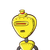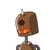# The perimeter of a rectangle is 64 cm. If its breadth is 15 cm, what is its length?

The perimeter of a rectangle is 64 cm. If its breadth is 15 cm, what is its length?

### 2 thoughts on “The perimeter of a rectangle is 64 cm. If its breadth is 15 cm, what is its length?”

1.Perimeter is P=2(L+B), and then 64=2(L+15) becomes 32=L+15 which is L=17.

To check does 64=17+10+17+10? Yes it does.

All in centimeters.

Therefore the length of the rectangle is 17 cm.

2.